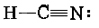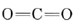# 7.4: Multiple Bonds and Molecular Shapes

 YouTube video links: Balloons, Hybrid Orbitals and Multiple Bonds Bonding and Balloons Lab

In a double bond, two electron pairs are shared between a pair of atomic nuclei. Despite the fact that the two electron pairs repel each other, they must remain between the nuclei, and so they cannot avoid each other. Therefore, for purposes of predicting molecular geometry, the two electron pairs in a double bond behave as one. They will, however, be somewhat “fatter” than a single electron-pair bond. For the same reason the three electron pairs in a triple bond behave as an “extra-fat” bond.

As an example of the multiple-bond rules, consider hydrogen cyanide, HCN. The Lewis structure isTreating the triple bond as if it were a single “fat” electron pair, we predict a linear molecule with an H―C―H angle of 180°. This is confirmed experimentally. Another example is formaldehyde, CH2O, whose Lewis structure isSince no lone pairs are present on C, the two H’s and the O should be arranged trigonally, with all four atoms in the same plane. Also, because of the “fatness” of the double bond, squeezing the C—H bond pairs together, we expect the H―C―H angle to be slightly less than 120°. Experimentally it is found to have the value of 117°.

Example $$\PageIndex{1}$$ : Shape

Predict the shape of the two molecules (a) nitrosyl chloride, NOCl, and (b) carbon dioxide, CO2.

Solution:

a) We must first construct a skeleton structure and then a Lewis diagram. Since N has a valence of 3, O a valence of 2, and Cl is monovalent, a probable structure for NOCl isCompleting the Lewis diagram, we findSince N has two bonds and one lone pair, the molecule must be angular. The O—N—Cl angle should be about 120°. Since the “fat” lone pair would act to reduce this angle while the “fat” double bond would tend to increase it, it is impossible to predict at this level of argument whether the angle will be slightly larger or smaller than 120°.

b) The Lewis structure of CO2 was considered in the previous chapter and found to beSince C has no lone pairs in its valence shell and each double bond acts as a fat bond pair, we conclude that the two O atoms are separated by 180° and that the molecule is linear.